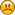Hello Guest

# Model rendering from +z to -z and rotating around a point doesnt showing.

• 2 Replies
• 635 Views####Joshi Elijah

•• 7##### Model rendering from +z to -z and rotating around a point doesnt showing.
« on: December 04, 2018, 06:46:09 »
Hi,

I am trying to rendering a model but it is projecting behind the camera, i am thinking of the problem being in the projection matrix from the link having the same problem https://stackoverflow.com/questions/23187262/lwjgl-projection-matrix-nothing-happens. I modified the projection matrix but still nothing happens, this is my projection matrix

Code: [Select]
` private static final float FOV = 70; private static final float NEAR_PLANE = 0.1f; private static final float FAR_PLANE = 10000;private void createProjectionMatrix() { float aspectRatio = (float) Display.getWidth() / (float) Display.getHeight(); float y_scale = (float) ((1f / Math.tan(Math.toRadians(FOV / 2f))) * aspectRatio); float x_scale = y_scale / aspectRatio; float frustum_length = FAR_PLANE - NEAR_PLANE; float bottom = -NEAR_PLANE * (float) Math.tan(Math.toRadians(FOV) / 2f); float top = -bottom; float left = aspectRatio * bottom; float right = -left; projectionMatrix = new Matrix4f(); projectionMatrix.m00 = x_scale; projectionMatrix.m11 = y_scale; projectionMatrix.m20 = (right+left) / (right-left); // projectionMatrix.m21 = (top+bottom) / (top-bottom); // projectionMatrix.m22 = -((FAR_PLANE + NEAR_PLANE) / frustum_length); projectionMatrix.m23 = -1; projectionMatrix.m32 = -((2 * NEAR_PLANE * FAR_PLANE) / frustum_length); projectionMatrix.m33 = 0; }`
Can someone help me ?this is important for me.####KaiHH

•• 219##### Re: Model rendering from +z to -z and rotating around a point doesnt showing.
« Reply #1 on: December 04, 2018, 18:58:04 »
The values for the projection matrix are 100% correct. Did you also take into account that such a projection matrix inverts the Z axis, transforming a vector from a right-handed coordinate system to OpenGL's expected left-handed clip space coordinate system? So, without any additional transformation, with your matrix everything will be visible with a Z value between -0.1 and -10000.0 (with sensible values for x and y of course).
If you multiply the vector (0, 0, -1, 1) by that matrix (with aspect ratio of 1) and divide by w, the result should be (0, 0, 0.8, 1), so: visible.####Joshi Elijah

•• 7##### Re: Model rendering from +z to -z and rotating around a point doesnt showing.
« Reply #2 on: December 05, 2018, 06:33:24 »
First of all thanks for your interest kaiHH, These are my vertices
Code: [Select]
`float[] vertices = { 1409.598f, -58.85f, -1471.946f, 1460.572f, -58.9047f, -1462.047f, 1408.506f, -20.5531f, -1471.137f }; int[] indices = { 0, 1, 2 };`
this is my transformation matrix and viewMatrix
Code: [Select]
`public static Matrix4f createTransformationMatrix(Vector3f translation, float rx, float ry, float rz, float scale) { Matrix4f matrix = new Matrix4f(); matrix.setIdentity(); Matrix4f.translate(translation, matrix, matrix); Matrix4f.rotate((float) Math.toRadians(rx), new Vector3f(1,0,0), matrix, matrix); Matrix4f.rotate((float) Math.toRadians(ry), new Vector3f(0,1,0), matrix, matrix); Matrix4f.rotate((float) Math.toRadians(rz), new Vector3f(0,0,1), matrix, matrix); Matrix4f.scale(new Vector3f(scale,scale,scale), matrix, matrix); return matrix; } public static Matrix4f createViewMatrix(Camera camera) { Matrix4f viewMatrix = new Matrix4f(); viewMatrix.setIdentity(); Matrix4f.rotate((float) Math.toRadians(camera.getPitch()), new Vector3f(1, 0, 0), viewMatrix, viewMatrix); Matrix4f.rotate((float) Math.toRadians(camera.getYaw()), new Vector3f(0, 1, 0), viewMatrix, viewMatrix); Vector3f cameraPos = camera.getPosition(); Vector3f negativeCameraPos = new Vector3f(-cameraPos.x,-cameraPos.y,-cameraPos.z); Matrix4f.translate(negativeCameraPos, viewMatrix, viewMatrix); return viewMatrix; }`you can find my complete project from this link https://www.dropbox.com/sh/ps9uu2clslke3qd/AADzqmNvCjslMipVvCMusuLCa?dl=0

Are my vertices too long for showing inside the camera ? Am working on this from a week now, Really happy if this gets sorted out.